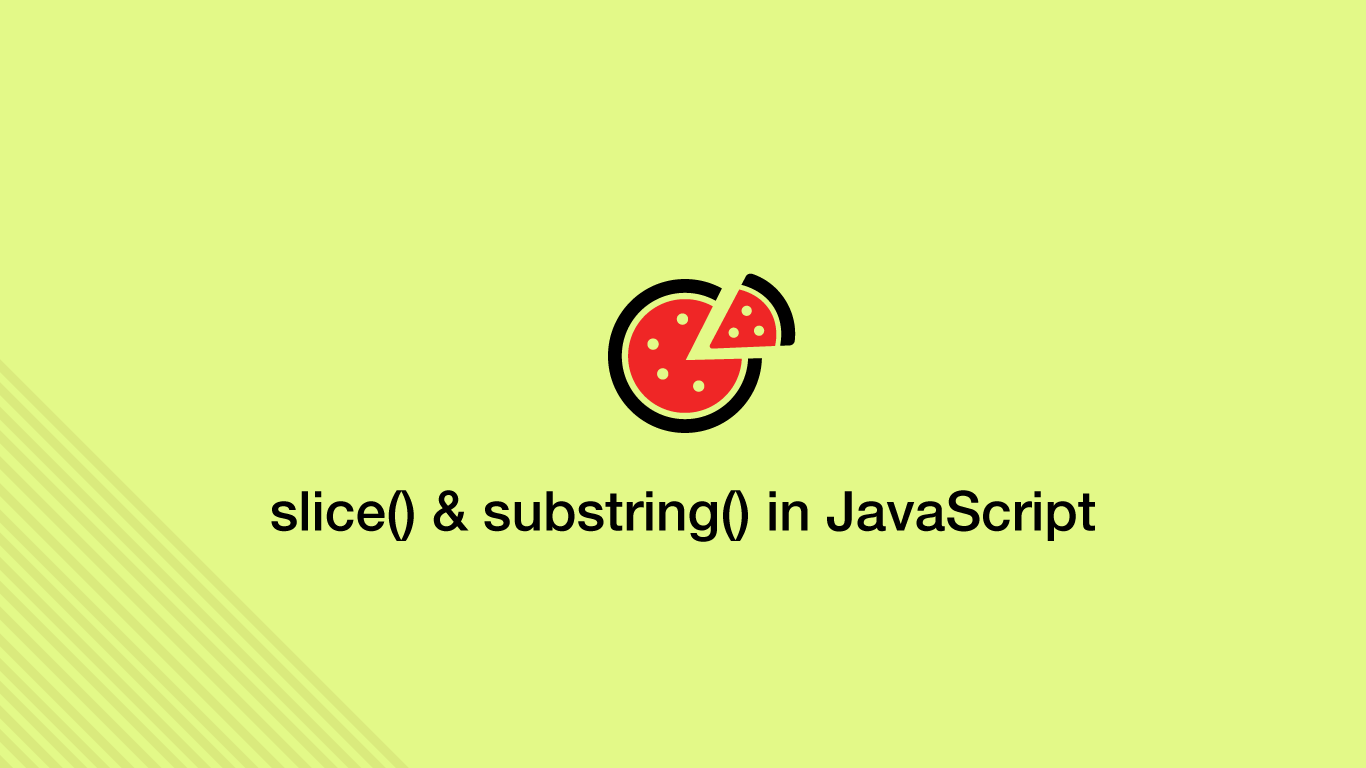# How to use slice() & substring() to Get Parts of a String in JavaScriptStrings in JavaScript are iterable and behave somewhat like arrays as each character in the string has its own index number. As a result, we can get a range of indexes from a string.

JavaScript provides three methods for getting parts of a string each of which has slightly different behaviours; `substring()`, `substr()` and `slice()`. In this tutorial, we will learn how to use these methods and what to do if you just need to get a single string character.

## Get an Index Of a String

To get one character from a string in JavaScript use `[]` (square brackets) containing the index of the character to get. String indexes start at `0` and count up.

``````var str = 'hello';

console.log(str);
``````
``````h
``````

## The substring() Method

The substring method will allow us to get a range of indexes from a string. To use it pass substring after the string variable using a `.` (dot). Inside the `()` (parenthesis) of `substring()` pass in two numerical arguments; the start index to include and the end index to exclude.

``````var str = 'cats'

var output = str.substring(1, 3);

console.log(output);
``````
``````at
``````

In cats a is at index `1` and s is at index `3`, since the behaviour of `substring()` is to exclude the end index we got `at`.

## The substr() Method

The `substr()` method works just like `substring()` except for two key differences; the end index is included and the start index can be negative. Let's use the same example as above, this time with `substr()`.

``````var str = 'catsy'

var output = str.substr(1, 3);

console.log(output);
``````
``````ats
``````

Indeed the end index (`3`) was included so the result is `ats`.

## Using a Negative Index with substr() to Get Characters from the End of a String

Negative indexes can be used with `substr()` to get characters from the end of a string. In the example below, we will get the last four characters from the string `database`.

``````var str = 'database'

var output = str.substr(-4);

console.log(output);
``````
``````base
``````

## The slice() Method

`slice()` behaves in an almost identical way to `substring()` except you can use negative indexes and it won't “flip” the index arguments if the start index is greater than the end index.

``````var str = 'database'

var output = str.slice(1, 3);

console.log(output);
``````
``````at
``````

It is possible to get characters starting from the end of the string with `slice()` by using a negative index.

``````var str = 'database'

var output = str.slice(-4);

console.log(output);
``````
``````base
``````

## How to Check if a String Contains a Substring

To check if a string contains a substring use the `includes()` method, passing the substring to look for as the first argument. `includes()` returns `true` or `false`.

``````var str = 'the quick brown fox'

var output = str.includes('fox');

console.log(output);
``````
``````true
``````

It is also possible to check if a substring exists after an index by passing the start index as the second argument.

``````var str = 'the quick brown fox'

var output = str.includes('quick', 10);

console.log(output);
``````
``````false
``````

## Conclusion

You now know how to get parts of a string using three different substring methods and how those methods behave slightly different from one another. You also know how to check if substrings exist in a string using the includes() method.